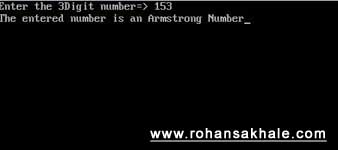# Armstrong Number Program

1/27/2012 code-examplecpp

### # Summary

Armstrong numbers also known as narcissistic numbers.

Armstrong numbers are the sum of their own digits to the power of the number of digits.

``````153 = (1*1*1) + (5*5*5) + (3*3*3)
``````
1

In this program will be find a 3 Digit number being entered by user is an Armstrong number or not.

For more details on Armstrong Number visit here (opens new window).

### # Screenshot### # Code

This program is hardcoded to 3 digits only

``````#include "stdio.h"
#include "conio.h"

void main()
{
int num;
int x,y,z;
// x resembles => Units Place
// y resembles => Thousands Place
// z resembles => Hundreds Place

clrscr();

printf("Enter the 3Digit number=> ");
scanf("%d", &num); //store the value in num

// Separated the number in terms of places

x = num % 10;
y = num / 100;
z = (num / 10) % 10;

// Cubing each place

x = x * x * x;
y = y * y * y;
z = z * z * z;

if(num == (x+y+z))
{
printf("The entered number is an Armstrong Number");
}
else
{
printf("It is not an Armstrong Number");
}
getch();
}
``````
1
2
3
4
5
6
7
8
9
10
11
12
13
14
15
16
17
18
19
20
21
22
23
24
25
26
27
28
29
30
31
32
33
34
35
36
37
38